# Kirchoff's Laws Series-Parallel Question

Status
Not open for further replies.

#### Ryan Thornwell

##### New Member
So here is the circuit, I am trying to figure current and volt drop through resistors.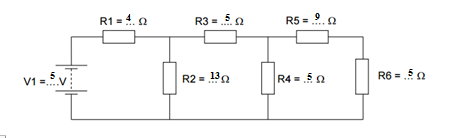I've got the circuit down to this by combining parallel and series. Also worked out total current.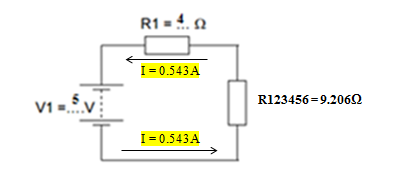I have this table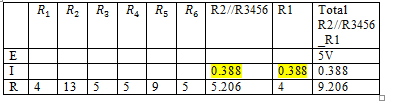Would I start by going back to the original circuit and first calculate E = I * R5//R6_R4? then do the same for each branch in the circuit?

#### MikeMl

##### Well-Known Member
Doesn't the total current (0.543106A) flow in R1?

If you know the current in R1, then you should be able to calculate the voltage at the node where R1, R2, and R3 connect. Similarly, you can work your way to the right progressively for the remaining nodes. I would label them...

Your calculation of the magnitude of the current from the voltage source is correct, but I do not see where you got the values you put in the table.

Also, if you ever hope to get a real job in the Electronics Industry, then the current in R1 flows from left to right...

Last edited:

#### Ryan Thornwell

##### New Member
If you know the current in R1, then you should be able to calculate the voltage at the node where R1, R2, and R3 connect. Similarly, you can work your way to the right progressively for the remaining nodes. I would label them...

Your calculation of the current from the voltage source is correct, but you screwed-up when filling in the table.

Doesn't the total current (0.543106A) flow in R1?

Also, if you ever hope to get a real job in the Electronics Industry, then the current in R1 flows from left to right...
So this is the first node right?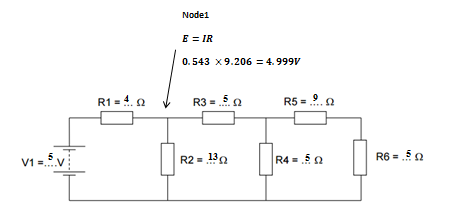Also fixed the table and current direction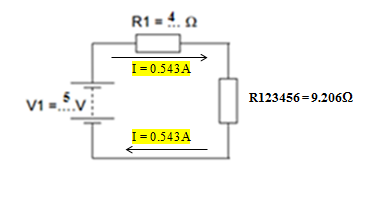Last edited:

#### Gasboss775

##### Member
Also, if you ever hope to get a real job in the Electronics Industry, then the current in R1 flows from left to right...
Speaking of this, there is another electronics site on the internet that insists on using the current flowing from negative to positive convention. I thought that issue had been settled years ago.

#### MikeMl

##### Well-Known Member
Speaking of this, there is another electronics site on the internet that insists on using the current flowing from negative to positive convention. I thought that issue had been settled years ago.
One of the reasons that I don't post there anymore...

Science, Education, and Industry use Conventional current which defines current as 1 Coulomb of positive charge flowing past a point in the circuit every second.

I agree with Wiki's definition of the convention:•spec and Gasboss775

#### The Electrician

##### Active Member
So this is the first node right?Also fixed the table and current directionThe equivalent resistance of 9.206 ohms appears at the left end of R1, not the right end.

#### Ryan Thornwell

##### New Member
Note that your equivalent circuit is not correct:

View attachment 97051

Study this:

View attachment 97049

Do you understand why I put the current source where I did, and how that defines V(b)?
My equivalent circuit is wrong because it gives the wrong current right?

You put the current source there because there the current will flow through the node?

#### Ryan Thornwell

##### New Member
Can't see where I've gone wrong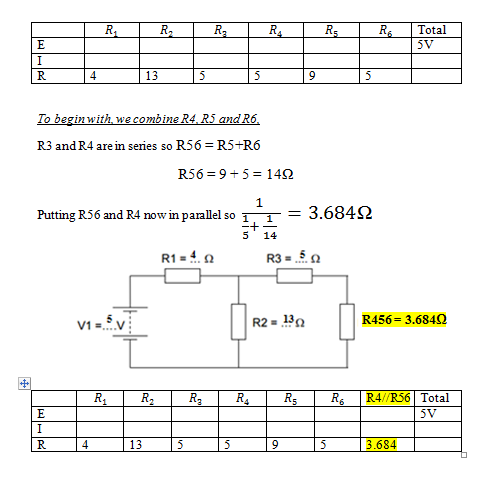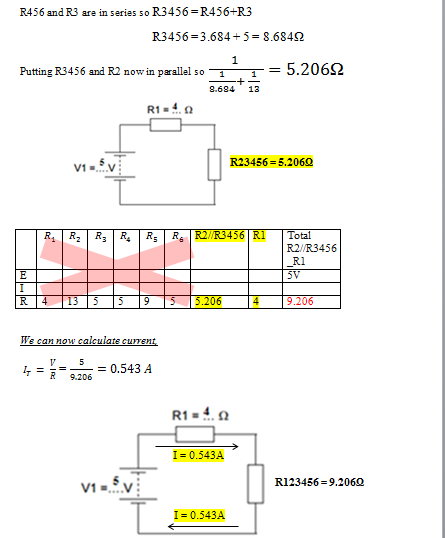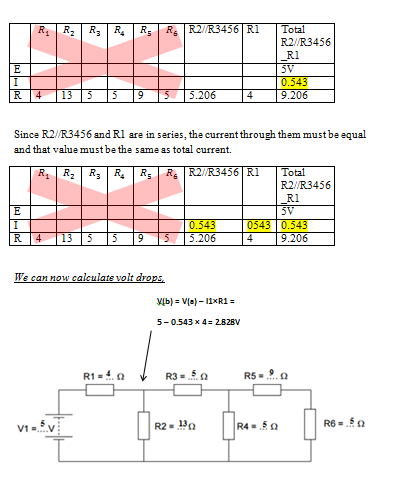#### MikeMl

##### Well-Known Member
My equivalent circuit is wrong because it gives the wrong current right?
Correct.

You put the current source there because there the current will flow through the node?
To say it a bit better: The 0.543A current source forces the current in R1 (and everywhere else in the circuit) to be the same as you already correctly calculated...

Note that you can arrive at V(b) by subtracting (I*R1) from 5.000V, as I tried to show in post #4. I only put the current source there so the sim could solve for V(b) without knowing the equivalent of R23456.

#### Ryan Thornwell

##### New Member
Correct.

To say it a bit better: The 0.543A current source forces the current in R1 (and everywhere else in the circuit) to be the same as you already correctly calculated...

Note that you can arrive at V(b) by subtracting (I*R1) from 5.000V, as I tried to show in post #4. I only put the current source there so the sim could solve for V(b) without knowing the equivalent of R23456.
Okay so my equivalent resistance should be 3.486?

Could you send the file for the sim?

#### MikeMl

##### Well-Known Member
You should be able to do this with pencil and paper....

Here is my effort:

#### Attachments

• 116.7 KB Views: 76

#### Ryan Thornwell

##### New Member
I definitely went for the longer approachYou've helped my understanding a lot thanks

As for the currents of the remaining resistors, they're given right?

#### Ryan Thornwell

##### New Member
I definitely went for the longer approachYou've helped my understanding a lot thanks

As for the currents of the remaining resistors, they're given right?
How are I
Here is the verification of the reduction steps:

e.g., compare V(b), V(b1) and V(b2)

View attachment 97062
How does I (R4) for instance = 0.239?

If its just I = V/R, then I (R4) = 2.298/3.684 = 0.624

#### MikeMl

##### Well-Known Member
...How does I (R4) for instance = 0.239?

If its just I = V/R, then I (R4) = 2.298/3.684 = 0.624
I(R4)=V(c)/R4 = 1.19958/5 = 0.239916, which agrees with the tabulated value of I(R4). The current into node C via R3 equals the sum of the currents that flow to ground via R4 and R5+R6, or I(R3) = I(R4) + I(R5). We can find the current through the R5 branch the same way. I(R5) = V(c)/(R5+R6) = 1.19958/(9+5) = 0.0856842, which matches the tabulated values for I(R5) and I(R6). The sum of I(R4) and I(R5) = 0.32560, which matches the tabulated value of I(R3).

KCL works for every node. Ohms Law works for every resistor...

#### Ratchit

##### Well-Known Member
So here is the circuit, I am trying to figure current and volt drop through resistors.I've got the circuit down to this by combining parallel and series. Also worked out total current.I have this tableWould I start by going back to the original circuit and first calculate E = I * R5//R6_R4? then do the same for each branch in the circuit?
There are a zillion ways to do this problem. Some of the ways are by loop, node, or branch analysis. Another way is the current divider law. For instance, the current present in R4 is I(R3) * (R5 + R6)/(R5+R6+R4). Since you already calculated the series-parallel values up the chain, they should be available for you to use.

Ratch

•spec

#### Ratchit

##### Well-Known Member
Speaking of this, there is another electronics site on the internet that insists on using the current flowing from negative to positive convention. I thought that issue had been settled years ago.
Why not name it? "All about Circuits", right? Yes, they are a rather quirky site, aren't they? They seem to think that current direction should be dependent on the polarity of the charge carriers. That means they have to think one way when they calculate electron flow in wires, and double-think reverse when they calculate hole current in semiconductors. It is much better to use the mathematical convention of assuming charge carriers are positive and charge flow is positive when it comes from the positive terminal of the voltage source. Then, if necessary to find the real direction of the current carriers, just reverse direction if the charge carriers are negative. Also, ammeters and semiconductors are marked using the mathematical convention. Too bad LTSpice is backwards.

Ratch

•spec

#### Ratchit

##### Well-Known Member

Look at the attached file in post #3. It shows both voltage sources as having a negative current.

Finally, look at the attached file documentation. In figure "a" it shows the current in the opposite direction to the mathematical convention. The descriptive paragraph in the middle of the page also describes the current direction as being opposite to the mathematical convention.

Ratch

#### Attachments

• 328.2 KB Views: 54
•spec
Status
Not open for further replies.